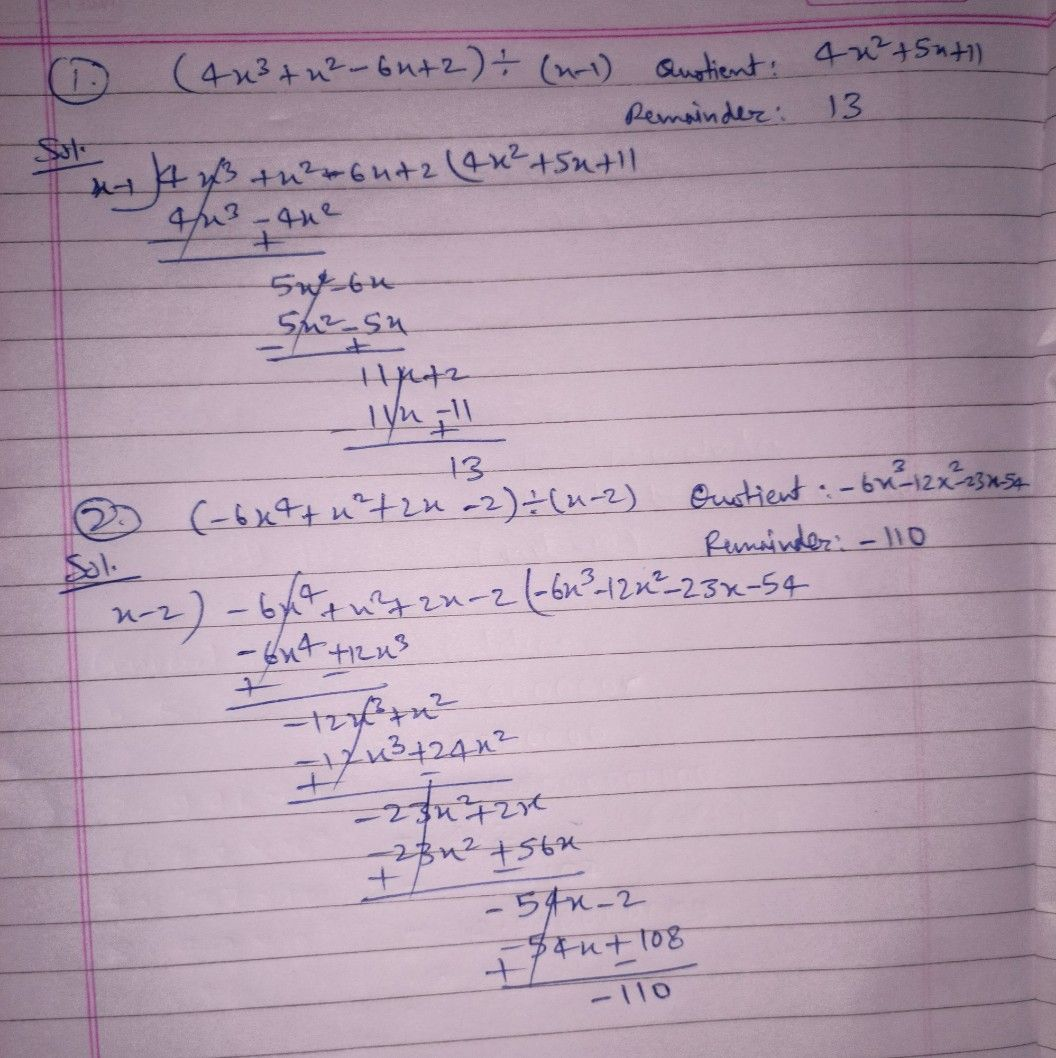Symbol
Problem$A$ $Usc$ synthetic $d$ division $to$ find the quotient and remainder in each of the $1$ $olo1$ $ing$ $nteyo4rcomPe$ $\left(4x^{3}+x^{2}-6x+2\right)+\left(x-1\right)$ solutions on $a$ separate sheet of paper. $O4oticmt$ $Rcmaindcr$ $2.\left(-6x^{4}+x^{2}+2x-2\right)+\left(x-2\right)$ $O4oticnt$ $Rcmainder$
10th-13th grade
Other
Search count: 114
SolutionQanda teacher - SHIVRAJcheck it dear ??
if it's helpful then evaluate the answer pleaseStudent
Thank you